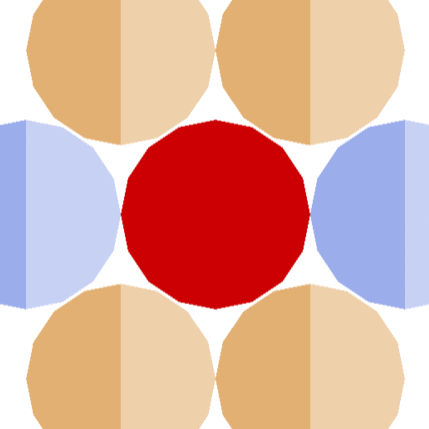DNA-Dave, the Transcription Translation Robot Educator

DNA-Dave is striving to be the best tool to teach kids molecular biology concepts. The key word is "striving."

IntermediateWork in progressOver 4 days1,835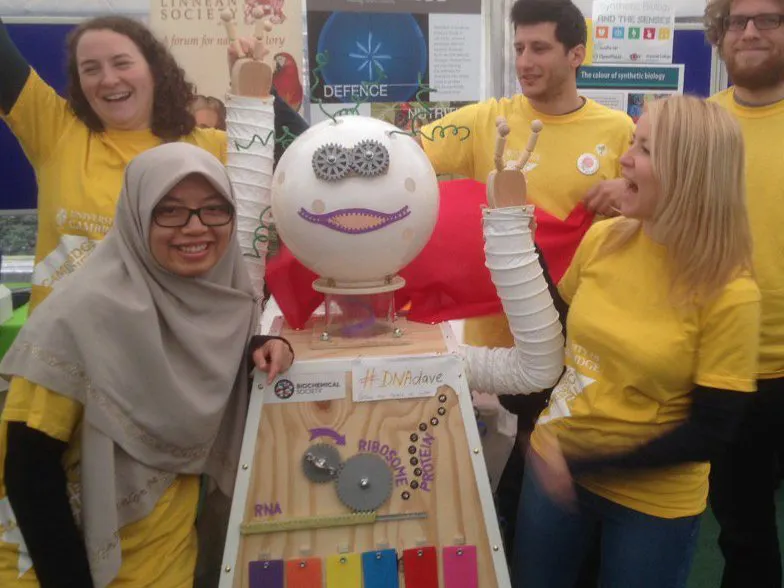Things used in this project

Hardware components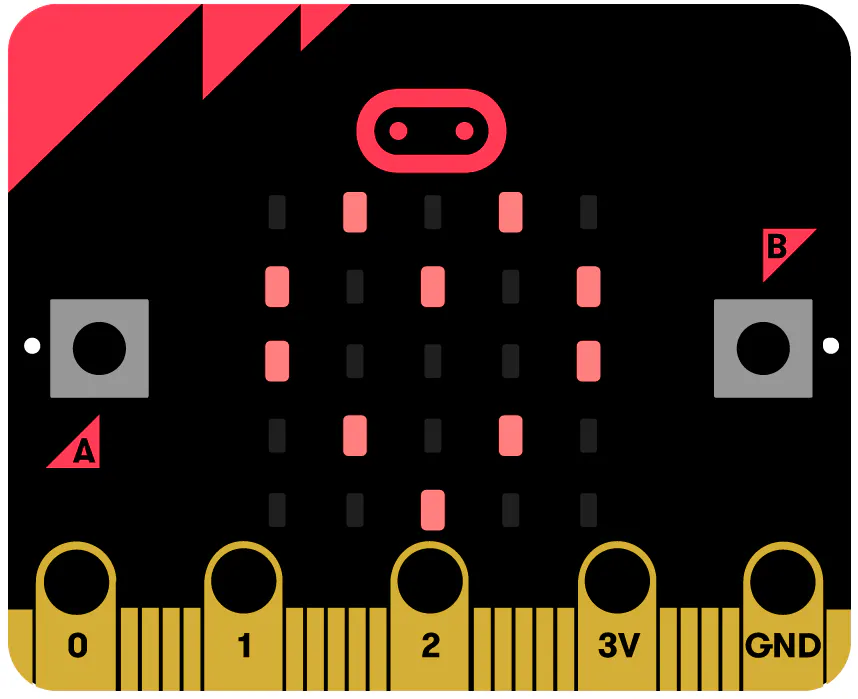BBC micro:bit board
×1
 ELECFREAKS octopus:bit
×1
×1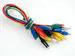Alligator Clips
×1Arduino UNO
×1

Software apps and online servicesMicrosoft MakeCode

Custom parts and enclosures

eDrawings file of all of DNA Dave's components. We suggest that you download solidworks eDrawings Viewer (which is free!).

Schematics

DNA-Dave_Arduino

fritzing schematic file

DNA-Dave_Arduino_png

schematic png file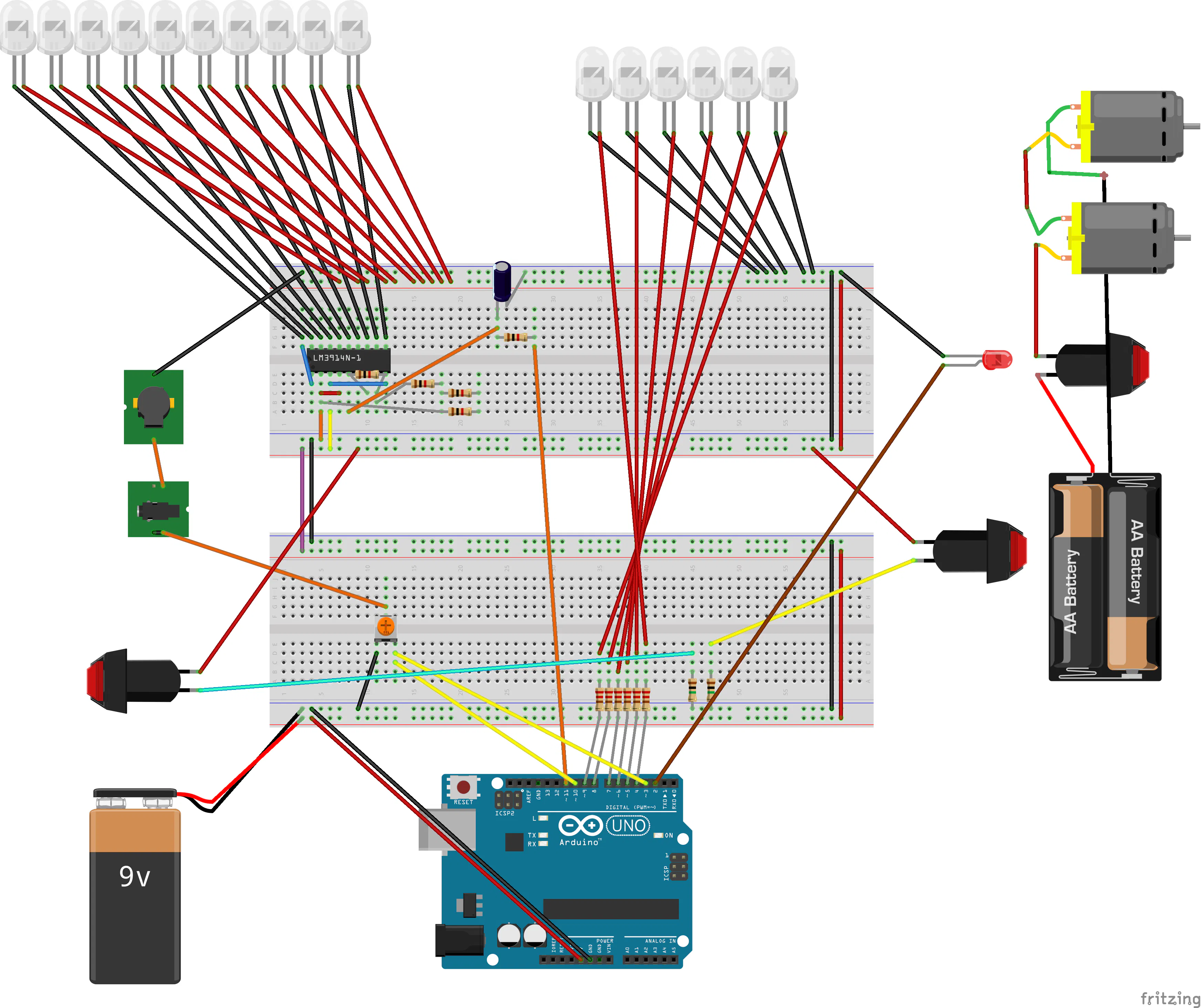DNA-Dave_microbit

fritzing diagram of microbit version of DNA-Dave. simpler wiring and code than the arduino version

DNA-Dave_microbit_diagram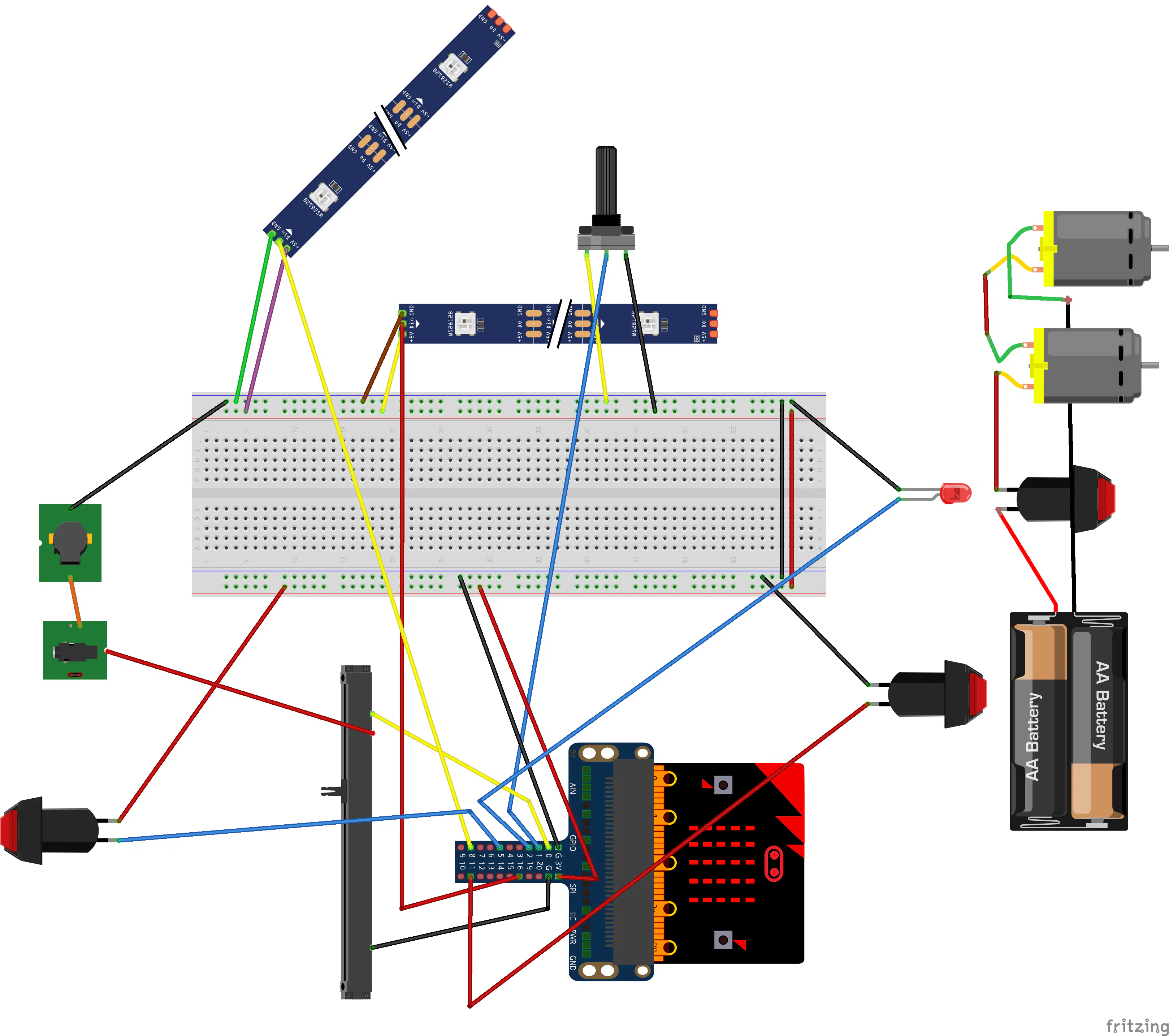Code

miniDave_microbit_DNA_translator

JavaScript
this is Javascript MakeCode code for a microbit connected to a strip of individually addressable LEDs, where you can input and visualise a DNA sequence, then translate it into an amino-acid sequence! Just paste it into the Javascript Window of MakeCode online editor. The AminoAcid color scheme is similar to RasMol amino color scheme, itself similar to Shapely scheme.
function transcribe_and_translate_the_DNA_string () {
RNA_string = []
AminoAcid_string = []
for (let index = 0; index <= DNA_string.length; index++) {
if (DNA_string[index] == "t") {
RNA_string.insertAt(index, "u")
} else {
RNA_string.insertAt(index, DNA_string[index])
}
}
number_of_triplets = RNA_string.length / 3
for (let index2 = 0; index2 <= number_of_triplets; index2++) {
RNA_triplet_position_1 = RNA_string.shift()
RNA_triplet_position_2 = RNA_string.shift()
RNA_triplet_position_3 = RNA_string.shift()
RNA_triplet = "" + RNA_triplet_position_1 + RNA_triplet_position_2 + RNA_triplet_position_3
AminoAcid = Universal_Genetic_Code_squashed[Universal_Genetic_Code_squashed.indexOf(RNA_triplet) + 1]
AminoAcid_string.push(AminoAcid)
}
}
input.onButtonPressed(Button.B, function () {
if (tilt_state == 1) {
DNA_string.push("g")
basic.showLeds(`
. # # # .
# . . . .
# . # # .
# . . # .
. # # # .
`)
} else {
DNA_string.push("a")
basic.showLeds(`
. # # . .
# . . # .
# . . # .
# # # # .
# . . # .
`)
}
})
input.onButtonPressed(Button.A, function () {
if (tilt_state == 0) {
DNA_string.push("t")
basic.showLeds(`
# # # # #
. . # . .
. . # . .
. . # . .
. . # . .
`)
} else {
DNA_string.push("c")
basic.showLeds(`
. # # # .
# . . . .
# . . . .
# . . . .
. # # # .
`)
}
})
input.onGesture(Gesture.Shake, function () {
if (translate_state == 0) {
translate_state = 1
strip.clear()
strip.show()
basic.showString("Protein")
} else {
translate_state = 0
basic.showString("DNA")
strip.clear()
strip.show()
}
})
input.onGesture(Gesture.TiltRight, function () {
tilt_state = 1
basic.showLeds(`
# # . . .
# . # # #
# # # . .
. . # . #
. . # # #
`)
})
input.onButtonPressed(Button.AB, function () {
DNA_string = []
basic.showLeds(`
# . . . #
. # . # .
. . # . .
. # . # .
# . . . #
`)
strip.clear()
})
input.onGesture(Gesture.TiltLeft, function () {
tilt_state = 0
basic.showLeds(`
# # # . .
. # . # .
. # # . #
. . # # #
. . # . #
`)
})
let position = 0
let AminoAcid = ""
let RNA_triplet = ""
let RNA_triplet_position_3 = ""
let RNA_triplet_position_2 = ""
let RNA_triplet_position_1 = ""
let number_of_triplets = 0
let RNA_string: string[] = []
let Universal_Genetic_Code_squashed: string[] = []
let AminoAcid_string: string[] = []
let DNA_string: string[] = []
let translate_state = 0
let tilt_state = 0
let strip: neopixel.Strip = null
strip = neopixel.create(DigitalPin.P0, 40, NeoPixelMode.RGB)
tilt_state = 0
translate_state = 0
DNA_string = []
AminoAcid_string = []
basic.showString("DNA!")
Universal_Genetic_Code_squashed = ["uuu", "phe", "uuc", "phe", "uua", "leu", "uug", "leu", "ucu", "ser", "ucc", "ser", "uca", "ser", "ucg", "ser", "uau", "tyr", "uac", "tyr", "uaa", "stop", "uag", "stop", "ugu", "cys", "ugc", "cys", "uga", "stop", "ugg", "trp", "cuu", "leu", "cuc", "leu", "cua", "leu", "cug", "leu", "ccu", "pro", "ccc", "pro", "cca", "pro", "ccg", "pro", "cau", "his", "cac", "his", "caa", "gln", "cag", "gln", "cgu", "arg", "cgc", "arg", "cga", "arg", "cgg", "arg", "auu", "ile", "auc", "ile", "aua", "ile", "aug", "met", "acu", "thr", "acc", "thr", "aca", "thr", "acg", "thr", "aau", "asn", "aac", "asn", "aaa", "lys", "aag", "lys", "agu", "ser", "agc", "ser", "aga", "arg", "agg", "arg", "guu", "val", "guc", "val", "gua", "val", "gug", "val", "gcu", "ala", "gcc", "ala", "gca", "ala", "gcg", "ala", "gau", "asp", "gac", "asp", "gaa", "glu", "gag", "glu", "ggu", "gly", "ggc", "gly", "gga", "gly", "ggg", "gly"]
basic.forever(function () {
position = -1
if (translate_state == 0) {
for (let deoxyribonucleotide of DNA_string) {
position += 1
if (deoxyribonucleotide == "a") {
strip.setPixelColor(position, neopixel.colors(NeoPixelColors.Blue))
} else if (deoxyribonucleotide == "t") {
strip.setPixelColor(position, neopixel.colors(NeoPixelColors.Green))
} else if (deoxyribonucleotide == "c") {
strip.setPixelColor(position, neopixel.colors(NeoPixelColors.Orange))
} else if (deoxyribonucleotide == "g") {
strip.setPixelColor(position, neopixel.colors(NeoPixelColors.Red))
}
}
} else {
transcribe_and_translate_the_DNA_string()
for (let AminoAcid2 of AminoAcid_string) {
position += 1
if (AminoAcid2 == "ala") {
strip.setPixelColor(position, neopixel.rgb(10, 10, 10))
} else if (AminoAcid2 == "arg") {
strip.setPixelColor(position, neopixel.rgb(40, 10, 235))
} else if (AminoAcid2 == "asn") {
strip.setPixelColor(position, neopixel.rgb(0, 150, 255))
} else if (AminoAcid2 == "asp") {
strip.setPixelColor(position, neopixel.rgb(255, 0, 0))
} else if (AminoAcid2 == "cys") {
strip.setPixelColor(position, neopixel.rgb(255, 200, 0))
} else if (AminoAcid2 == "glu") {
strip.setPixelColor(position, neopixel.rgb(230, 10, 10))
} else if (AminoAcid2 == "gln") {
strip.setPixelColor(position, neopixel.rgb(0, 255, 150))
} else if (AminoAcid2 == "gly") {
strip.setPixelColor(position, neopixel.rgb(235, 235, 235))
} else if (AminoAcid2 == "his") {
strip.setPixelColor(position, neopixel.rgb(255, 0, 255))
} else if (AminoAcid2 == "ile") {
strip.setPixelColor(position, neopixel.rgb(15, 90, 15))
} else if (AminoAcid2 == "leu") {
strip.setPixelColor(position, neopixel.rgb(0, 255, 0))
} else if (AminoAcid2 == "lys") {
strip.setPixelColor(position, neopixel.rgb(0, 0, 255))
} else if (AminoAcid2 == "met") {
strip.setPixelColor(position, neopixel.rgb(200, 255, 5))
} else if (AminoAcid2 == "phe") {
strip.setPixelColor(position, neopixel.rgb(0, 0, 50))
} else if (AminoAcid2 == "pro") {
strip.setPixelColor(position, neopixel.rgb(200, 100, 40))
} else if (AminoAcid2 == "ser") {
strip.setPixelColor(position, neopixel.rgb(255, 100, 0))
} else if (AminoAcid2 == "thr") {
strip.setPixelColor(position, neopixel.rgb(255, 50, 0))
} else if (AminoAcid2 == "trp") {
strip.setPixelColor(position, neopixel.rgb(180, 40, 60))
} else if (AminoAcid2 == "tyr") {
strip.setPixelColor(position, neopixel.rgb(10, 0, 40))
} else if (AminoAcid2 == "val") {
strip.setPixelColor(position, neopixel.rgb(10, 200, 10))
} else if (AminoAcid2 == "stop") {
strip.setPixelColor(position, neopixel.colors(NeoPixelColors.Black))
}
}
}
strip.show()
})

miniDave_microbit_DNA_only_visualiser_simple

JavaScript
This is MakeCode JavaScript code to use with a microbit that is connected to a strip/string of individually addressable LEDs. The user can input a DNA sequence using the microbit and see it in the strip of LEDs! It uses the neopixel extension.
input.onButtonPressed(Button.A, function () {
if (tilt_state == 0) {
DNA_string.push("t")
basic.showLeds(`
# # # # #
. . # . .
. . # . .
. . # . .
. . # . .
`)
} else {
DNA_string.push("c")
basic.showLeds(`
. # # # .
# . . . .
# . . . .
# . . . .
. # # # .
`)
}
})
input.onButtonPressed(Button.B, function () {
if (tilt_state == 1) {
DNA_string.push("g")
basic.showLeds(`
. # # # .
# . . . .
# . # # .
# . . # .
. # # # .
`)
} else {
DNA_string.push("a")
basic.showLeds(`
. # # . .
# . . # .
# . . # .
# # # # .
# . . # .
`)
}
})
input.onGesture(Gesture.TiltLeft, function () {
tilt_state = 0
basic.showLeds(`
# # # . .
. # . # .
. # # . #
. . # # #
. . # . #
`)
})
input.onGesture(Gesture.TiltRight, function () {
tilt_state = 1
basic.showLeds(`
# # . . .
# . # # #
# # # . .
. . # . #
. . # # #
`)
})
input.onButtonPressed(Button.AB, function () {
DNA_string = []
basic.showLeds(`
# . . . #
. # . # .
. . # . .
. # . # .
# . . . #
`)
strip.clear()
})
let position = 0
let DNA_string: string[] = []
let tilt_state = 0
let strip: neopixel.Strip = null
strip = neopixel.create(DigitalPin.P16, 150, NeoPixelMode.RGB)
tilt_state = 0
DNA_string = []
basic.showString("DNA!")
basic.forever(function () {
position = -1
for (let deoxyribonucleotide of DNA_string) {
position += 1
if (deoxyribonucleotide == "a") {
strip.setPixelColor(position, neopixel.colors(NeoPixelColors.Red))
} else if (deoxyribonucleotide == "t") {
strip.setPixelColor(position, neopixel.colors(NeoPixelColors.Orange))
} else if (deoxyribonucleotide == "c") {
strip.setPixelColor(position, neopixel.colors(NeoPixelColors.Blue))
} else if (deoxyribonucleotide == "g") {
strip.setPixelColor(position, neopixel.colors(NeoPixelColors.Indigo))
}
}
strip.show()
})

DNA-Dave_microbit_javascriptFromMakeCode

JavaScript
goto Makecode website and paste it into the javascript part of a project
function Glissanto (note1: number, note2: number, rate: number) {
if (note1 < note2) {
i = note1
while (i < note2) {
music.ringTone(i)
control.waitMicros(rate)
i += 2
}
} else {
i = note1
while (i > note2) {
music.ringTone(i)
control.waitMicros(rate)
i += -2
}
}
music.rest(0)
}
function Tremolo (note: number, length: number, rate: number) {
note_duration = length / rate
pauseBetweenNotes = rate * (1 + rate / length)
for (let index = 0; index <= rate; index++) {
music.playTone(note, note_duration)
music.rest(pauseBetweenNotes)
}
music.rest(0)
}
input.onButtonPressed(Button.A, function () {
greenArmpitButtonSound()
control.waitMicros(1000)
})
input.onButtonPressed(Button.B, function () {
redArmpitButtonSound()
control.waitMicros(1000)
})
function success_sound () {
for (let index2 = 0; index2 <= Math.randomRange(1, 6); index2++) {
for (let index22 = 0; index22 <= 1000; index22++) {
music.ringTone(1000 + index22)
control.waitMicros(300)
}
}
music.rest(music.beat(BeatFraction.Whole))
music.stopMelody(MelodyStopOptions.All)
}
function angry_Dave_sound () {
for (let index3 = 0; index3 <= 4; index3++) {
for (let index23 = 0; index23 <= 1000; index23++) {
music.ringTone(1000 - index23)
control.waitMicros(300)
}
}
music.rest(music.beat(BeatFraction.Whole))
music.stopMelody(MelodyStopOptions.All)
}
function dispense_protein () {
which_protein = Math.randomRange(0, strip2.length() - 1)
strip2.setPixelColor(which_protein, neopixel.rgb(Math.randomRange(0, 255), Math.randomRange(0, 255), Math.randomRange(0, 255)))
strip2.setPixelColor(Math.abs((which_protein - 1) % strip2.length()), neopixel.rgb(Math.randomRange(0, 255), Math.randomRange(0, 255), Math.randomRange(0, 255)))
strip2.show()
for (let index4 = 0; index4 <= 9 * strip2.length() - 1; index4++) {
strip2.rotate(1)
strip2.show()
music.ringTone(index4 * index4)
control.waitMicros(index4 * 7000)
}
strip2.clear()
strip2.setPixelColor(which_protein, neopixel.colors(NeoPixelColors.Green))
strip2.show()
success_sound()
control.waitMicros(6000000)
strip.clear()
strip.show()
control.waitMicros(2000000)
strip2.clear()
strip2.show()
}
function redArmpitButtonSound () {
rand = Math.randomRange(1, 4)
if (rand == 1) {
Glissanto(784, 392, 0)
Glissanto(392, 784, 0)
control.waitMicros(100000)
Glissanto(784, 392, 0)
Glissanto(392, 784, 0)
}
if (rand == 2) {
Glissanto(1400, 1000, 0)
Glissanto(1200, 800, 0)
Glissanto(1000, 6000, 0)
control.waitMicros(300000)
Tremolo(440, 30, 3)
control.waitMicros(100000)
Tremolo(659, 30, 12)
}
if (rand > 2) {
Glissanto(784, 392, 0)
control.waitMicros(300000)
Glissanto(784, 392, 0)
Glissanto(784, 196, 0)
}
}
function greenArmpitButtonSound () {
rand = Math.randomRange(1, 5)
if (rand == 1) {
Glissanto(784, 392, 0)
control.waitMicros(300000)
Glissanto(880, 220, 0)
control.waitMicros(100000)
Tremolo(175, 30, 3)
control.waitMicros(100000)
Tremolo(131, 30, 3)
control.waitMicros(100000)
Glissanto(131, 523, 0)
Glissanto(988, 392, 0)
}
if (rand == 2) {
Glissanto(500, 2000, 0)
control.waitMicros(500000)
Tremolo(800, 30, 3)
control.waitMicros(100000)
Tremolo(600, 30, 3)
control.waitMicros(100000)
Glissanto(300, 1700, 0)
Glissanto(600, 3000, 0)
}
if (rand == 3) {
Glissanto(6000, 200, 0)
control.waitMicros(500000)
Tremolo(1000, 30, 3)
control.waitMicros(100000)
Tremolo(600, 30, 3)
control.waitMicros(100000)
Tremolo(800, 30, 3)
control.waitMicros(100000)
Tremolo(400, 30, 3)
}
if (rand == 4) {
Glissanto(6000, 5500, 100)
control.waitMicros(100000)
Glissanto(6000, 5500, 100)
control.waitMicros(300000)
Glissanto(6000, 5500, 100)
control.waitMicros(100000)
Tremolo(1000, 30, 3)
control.waitMicros(10000)
Tremolo(600, 30, 3)
control.waitMicros(10000)
Tremolo(800, 30, 3)
control.waitMicros(10000)
Tremolo(400, 30, 3)
}
if (rand == 5) {
Glissanto(294, 2000, 0)
control.waitMicros(500000)
Tremolo(1760, 30, 3)
control.waitMicros(100000)
Tremolo(1396, 30, 3)
control.waitMicros(100000)
Glissanto(5000, 2500, 0)
Glissanto(5000, 2500, 0)
}
}
let which_LED = 0
let cog_average_before = 0
let cog_average = 0
let Dave_state = 0
let cog_voltage = 0
let rand = 0
let which_protein = 0
let pauseBetweenNotes = 0
let note_duration = 0
let i = 0
let strip2: neopixel.Strip = null
let strip: neopixel.Strip = null
strip = neopixel.create(DigitalPin.P8, 10, NeoPixelMode.RGB)
strip2 = neopixel.create(DigitalPin.P16, 6, NeoPixelMode.RGB)
let increment = 20
let dispensed_protein = 1
basic.forever(function () {
control.waitMicros(1000)
if (Dave_state == 0) {
strip.clear()
strip.show()
cog_average = 0
pins.digitalWritePin(DigitalPin.P2, 1)
if (cog_voltage < 20) {
pins.digitalWritePin(DigitalPin.P2, 0)
strip.clear()
strip2.clear()
strip.show()
strip2.show()
Dave_state = 1
cog_average_before = 20
}
} else if (Dave_state == 1) {
cog_average += (cog_voltage - cog_average) * 0.7
which_LED = Math.round(Math.map(cog_average, 0, 1023, 0, strip.length() + 2))
for (let index5 = 0; index5 <= which_LED; index5++) {
strip.setPixelColor(index5, neopixel.rgb(Math.randomRange(0, 255), Math.randomRange(0, 255), Math.randomRange(0, 255)))
}
strip.show()
if (cog_average > cog_average_before + increment) {
cog_average_before = cog_average
} else if (cog_average < cog_average_before - increment) {
Dave_state = 2
}
if (cog_average > 740) {
Dave_state = 3
}
} else if (Dave_state == 2) {
angry_Dave_sound()
Dave_state = 0
} else if (Dave_state == 3) {
success_sound()
dispense_protein()
Dave_state = 0
strip.clear()
strip.show()
}
})

DNA-Dave_Arduino

This is the code that runs on the arduino version of DNA-Dave

Credits

Ioannis Tamvakis

2 projects • 1 follower

sami.s

0 projects • 0 followers